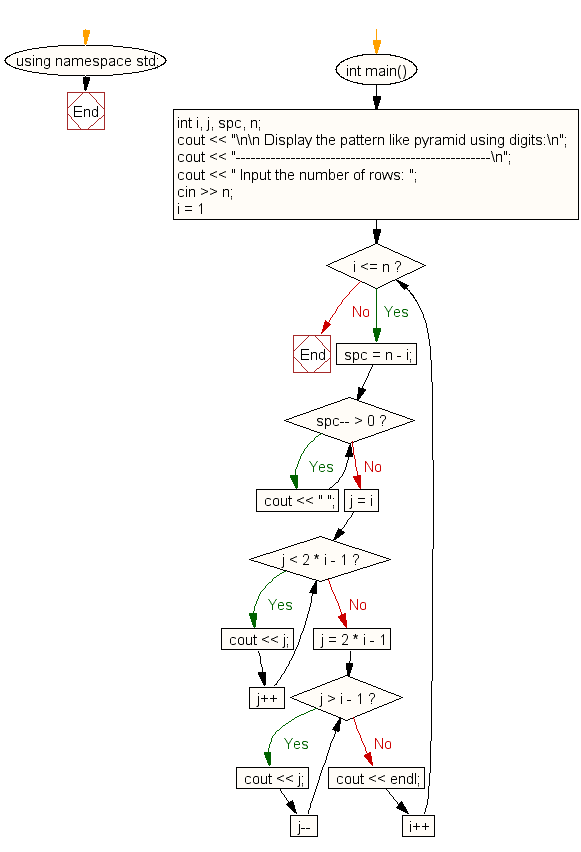﻿ C++ : Pyramid of digits as shown below for n number of lines# C++ Exercises: Print a pyramid of digits as shown below for n number of lines

## C++ For Loop: Exercise-49 with Solution

Write a program in C++ to print a pyramid of digits as shown below for n number of lines.

```    1
232
34543
4567654
567898765
```

Sample Solution:-

C++ Code :

``````#include <iostream>
using namespace std;

int main()
{
int i, j, spc, n;
cout << "\n\n Display the pattern like pyramid using digits:\n";
cout << "---------------------------------------------------\n";
cout << " Input the number of rows: ";
cin >> n;
for (i = 1; i <= n; i++)
{
spc = n - i;
while (spc-- > 0)
cout << " ";
for (j = i; j < 2 * i - 1; j++)
cout << j;
for (j = 2 * i - 1; j > i - 1; j--)
cout << j;
cout << endl;
}
}
``````

Sample Output:

``` Display the pattern like pyramid using digits:
---------------------------------------------------
Input the number of rows: 5
1
232
34543
4567654
567898765
```

Flowchart:C++ Code Editor:

Contribute your code and comments through Disqus.

What is the difficulty level of this exercise?

﻿